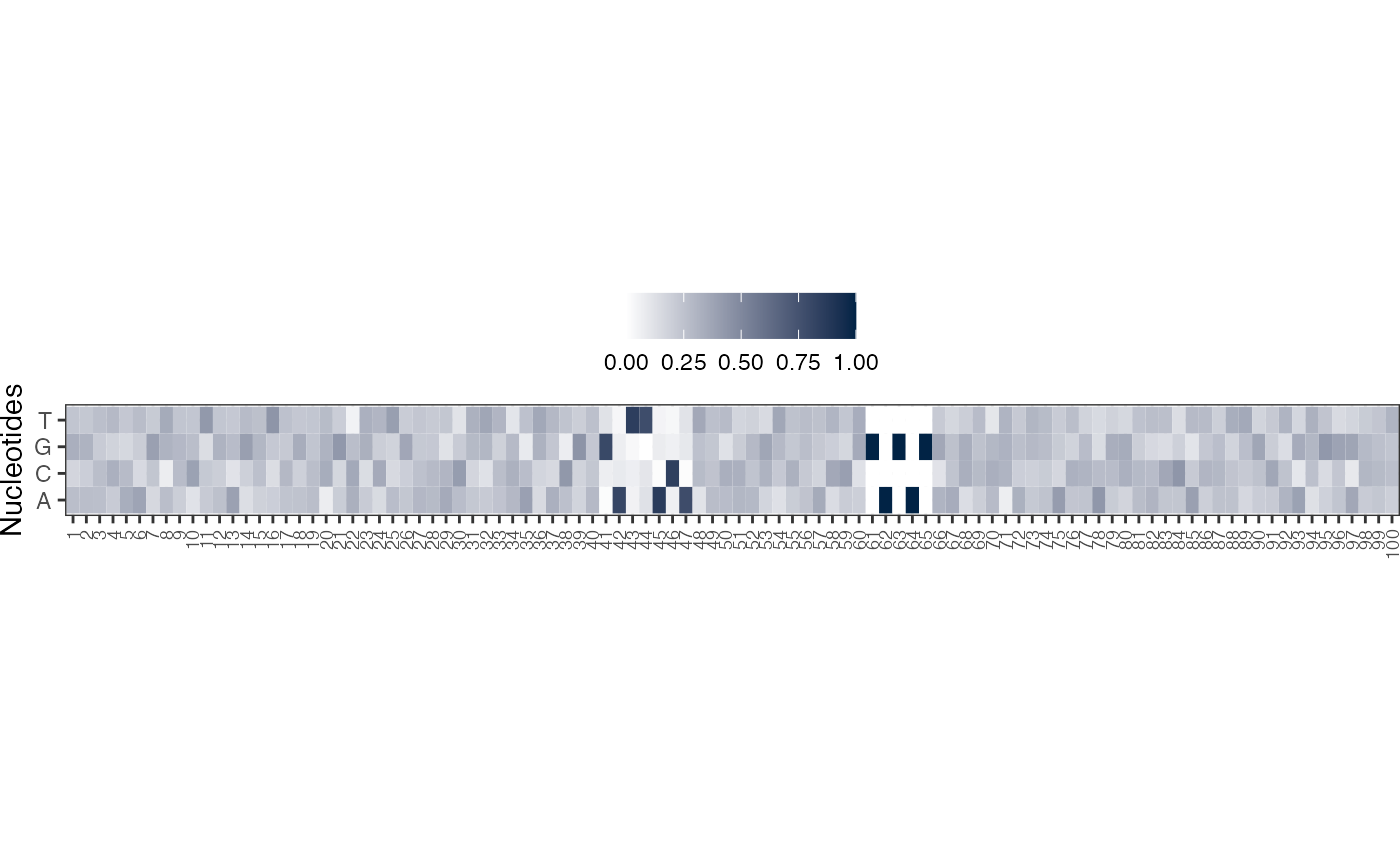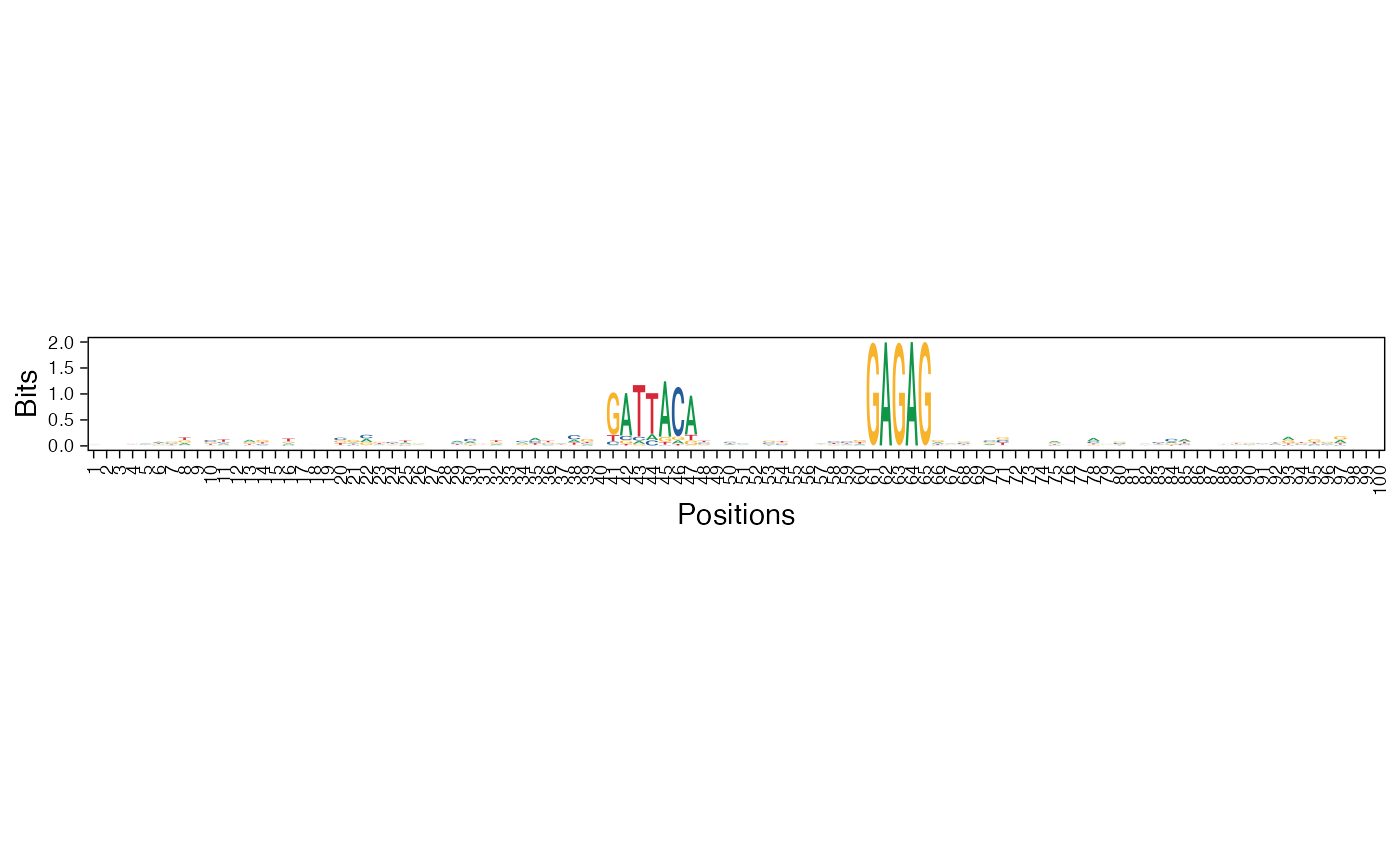The given position weight matrix is plotted as a heatmap or sequence logo

viz_pwm(
pwm_mat,
method = "heatmap",
pos_lab = NULL,
pdf_name = NULL,
fixed_coord = FALSE,
bits_yax = "full"
)

## Arguments

pwm_mat

Matrix (usually a PWM, but can be any non-normalized matrix) to be visualized. Rownames must be letters.

method

Character. Set this to 'heatmap' when plotting a heatmap, else you can set it to either of 'custom', 'bits', or 'probability' when you wish to visualize it as a sequence logo. Default is 'heatmap'.

pos_lab

Labels for sequence positions, should be of same length as that of the sequences. Default value is NULL, when the positions are labeled from 1 to the length of the sequences.

pdf_name

Name of the file which will be saved as PDF.

fixed_coord

Set this to TRUE to use a fixed aspect ratio for the plot. Default is FALSE.

bits_yax

Specify 'full' if the information content y-axis limits should be 0-2 or 'auto' for a suitable limit. The 'auto' setting adjusts the y-axis limits according to the maximum information content of the sequence logo. Default is 'full'.

## Value

A ggplot object so you can simply call print or save

on it later. If pdf_name is given, it is also saved and the ggplot2 object returned.

plot_ggseqlogo_of_seqs for visualizing a collection of sequences by their sequence logo.

Other visualization functions: viz_bas_vec(), viz_seqs_acgt_mat()

## Examples


package = "seqArchR", mustWork = TRUE))

pwm <- seqArchR::make_PWMs(get_clBasVec_m(res,iter=1)[,1],
add_pseudo_counts = FALSE, sinuc = FALSE)

viz_pwm(pwm_mat = pwm, method = "heatmap", fixed_coord = TRUE)viz_pwm(pwm_mat = pwm, method = "bits", fixed_coord = TRUE)
#> Scale for x is already present.
#> Adding another scale for x, which will replace the existing scale.